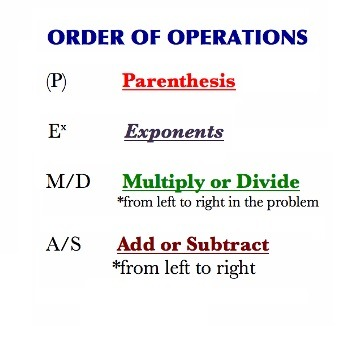# The order of math operations

When we perform computational actions in mathematics, we must keep in mind that there is a sequence that needs to be respected in order to do the calculation properly. Operations are divided into three degrees, and we start calculation by dealing with the third degree operations first. Because a single mathematical expression can be (but doesn’t have to be) made of different degree operations, we need to keep in mind two things:

• if an expression is made up of operations of the same degree, we resolve them from left to right.
• if an expression is made up of operations of different degrees, we resolve it by following the degree order.

So, this is the order of operations, with regard to the order of solving:

1. Exponents and roots (third degree operations)
2. Multiplication and division (second degree operations)
3. Addition and subtraction (first degree operations)

For example, let’s try solving this problem while respecting the order of operations, and then we’ll try it the other way around to see the difference.

#### Example 1.

$5 + 4 \cdot 2 = ?$

With respect to the order of operations:

$5 + 4 \cdot 2 = 5 + 8 = 13$

From left to right:

$5 + 4 \cdot 2 = 9 \cdot 2 = 18$

As you can see, the order of operations makes quite the difference. But, what if we want the addition to be performed before multiplication? Can we do that? Well, sure we can – if we surround the appropriate part with brackets.### Brackets

Brackets are not operations themselves, but symbols that are of vital importance to the order of operations. It’s because we can use brackets to override the proper order of operations.

Brackets are put around the parts of mathematical expressions we want to be solved first. There are three different types of brackets: round brackets or parentheses ( ), square brackets or hard brackets or just brackets [ ], and curly brackets or braces { }.

There are other types of brackets, but only these are used in the context of the order of operations. In mathematics, they always come in pairs – an opening bracket, which are {, [ or (, and the closing bracket, which are ), ], or }.

Brackets can be put around other brackets, thus creating so called nested brackets.  Because of the existence of nested brackets, and because there are three types available, there is an order both for solving and nesting brackets.

Brackets are nested this way:

1. The round brackets or parentheses are the first degree brackets and they do not go around any other brackets.
2. The square brackets are used when we want to create an expression that includes an expression that is already inside round brackets.
3. The curly brackets are used when we want to create an expression that includes an expression that is already inside square brackets.

So, an expression that uses all three types of brackets could look like this:

$4 + 18 \cdot {24 – 8 \cdot [(23-8) + 3 \cdot (32 + 4)] – 8 \cdot (23-4)}$

See? Now that we know the proper order of operations, the brackets give us precise instructions on how this problem should be solved in order to get to the correct result. And in what order are brackets solved? Well, we start from the inside, by solving the problems inside round brackets first, then work our way out. Like this:

Example:

$49 + 3 \cdot {24 – 2 \cdot [(23-8) + 3 \cdot (3 + 4)] – 8 \cdot (2 – 4)}$

$=49 + 3 \cdot {24 – 2 \cdot [15 + 3 \cdot 7] – 8 \cdot (-2)}$

$=49 + 3 \cdot {24 – 2 \cdot [15 + 21] – 8 \cdot (-2)}$

$=49 + 3 \cdot {24 – 2 \cdot 36 – 8 \cdot (-2)}$

$=49 + 3 \cdot {24 – 72 + 16}$

$= 49 + 3 \cdot (-32)$

$=49 – 96$

$=- 47$

Just for fun, try solving this problem just any old way and see what different results you would get.

Well, that’s all for our lesson on the order of operations. Since this is very important and can be a bit tough to master, feel free to practice some more using the worksheets below.